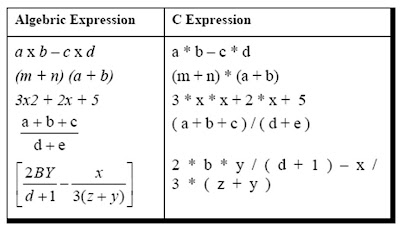Priority of Arthematic Operations in C Language Programming

The previous post of the blog about c programming deals with concept of mathematical conversions.

The priority or precedence in which the operations in an arithmetic statement are performed is called the hierarchy of operations. The hierarchy of commonly used operators is shown in Figure .General rules :

1 . Within parentheses the same hierarchy as mentioned in Figure 1.11 is operative. Also, if there are more than one set of parentheses, the operations within the innermost parentheses would be performed first, followed by the operations within the second innermost pair and so on.

2 . We must always remember to use pairs of parentheses. A careless imbalance of the right and left parentheses is a common error. Best way to avoid this error is to type ( ) and then type an expression inside it.

Examples :

1 . Determine the hierarchy of operations and evaluate the following expression:

i = 2 * 3 / 4 + 4 / 4 + 8 - 2 + 5 / 8

This can be done step by step as shown.

i = 6 / 4 + 4 / 4 + 8 - 2 + 5 / 8 operation: *
i = 1 + 4 / 4 + 8 - 2 + 5 / 8 operation: /
i = 1 + 1+ 8 - 2 + 5 / 8 operation: /
i = 1 + 1 + 8 - 2 + 0 operation: /
i = 2 + 8 - 2 + 0 operation: +
i = 10 - 2 + 0 operation: +
i = 8 + 0 operation : -
i = 8 operation: +

Note that 6 / 4 gives 1 and not 1.5. This so happens because 6 and 4 both are integers and therefore would evaluate to only an integer constant. Similarly 5 / 8 evaluates to zero, since 5 and 8 are integer constants and hence must return an integer value.

2 . Determine the hierarchy of operations and evaluate the following expression:

k = 3 / 2 * 4 + 3 / 8 + 3

Stepwise evaluation of this expression is shown below:

k = 3 / 2 * 4 + 3 / 8 + 3
k = 1 * 4 + 3 / 8 + 3 operation: /
k = 4 + 3 / 8 + 3 operation: *
k = 4 + 0 + 3 operation: /
k = 4 + 3 operation: +
k = 7 operation: +

All operators in C are ranked according to their precedence.

conversion of a general arithmetic statement to a C statement :

Here is the list of some simple examples which will give a outline about the conversion .Related posts :

IF STATEMENT

MULTIPLE STATEMENTS IN IF

IF AND ELSE

NESTED IF AND ELSE

BREAK

CONTINUE AND DO WHILE IN C LANGUAGE

SWITCH IN C PROGRAMMING

FUNCTIONS IN C PROGRAMMING

Functions and usage in C part two

Coding in C functions